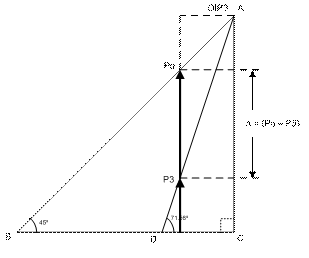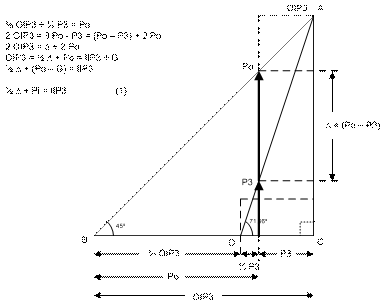Determining IIP3 of a DUT

[Proof of Identity:  1/2 ∆ + Pi = IIP3   --using geometry]

Syed M. Ahmed

The concept of Third-Order Intercept or IP3 in a gradual third- and higher-order response, may be better grasped and the relationships and definitions easily explained by rendering the problem to geometric representation as shown below.

The IP3 measurement is performed by carefully summing two frequency tones typically 1MHz apart and sufficient (equal) power level, and applying to the input of the DUT. The DUT output when viewed on a Spectrum Analyzer may look like the Fig:1 below. The fundamental input tones at higher power  flanked by third-order components usually at lower power level. Since the input power Pi is known the Power Gain (G) may be determined, one can then calculate the IIP3 using the well known identity: 1/2 ∆ + Pi = IIP3; where ∆ = (Po - P3), and may be measured directly from the Spectrum Analyzer.‡‡ The details of setting up bench hardware are described in the pdf version of this article and may be requested by e-mailing to the contact below.

Proof of Identity:  1/2 ∆ + Pi = IIP3   where  ∆ = (Po - P3)    --  (1)

In the above described setup, if the input power to the DUT is increased gradually, for example in 1dB steps, then the output tones rise at different rates. The 3rd order tone rising at 3x the rate of the fundamental, which rises at 1x or 1dB, for every 1dB rise of input power. The accompanying geometric figure captures this principle, where two right-triangles BAC and DAC, share a common side CA while the two slopes corresponding to 1x and 3x are represented by their hypotenuse BA and DA.Applying the properties of right-triangles, in triangle BAC the hypotenuse having slope 1x forces the sides BC and CA to be equal and the corresponding angles to be 45º. The inner triangle DAC, the hypotenuse DA is constructed with a slope 3x so that the other two sides DC and CA become fixed in that same ratio i.e. side CA is 3x of side DC, while the angle ADC is Tan-1 (AC/CD=3) or 71.56°. If the output power is depicted by a pair of vectors Po and P3 at an arbitrary distance past point D, then one can follow the outcome of gradual rise of input power to the DUT; by pushing to the right and growing the vectors Po and P3 at 1x and 3x rates until they both reach the same size and coincide with side CA at the point A, or point C along the base. Point A represents the Output Third-Order Intercept OIP3 which is a hypothetical point since the DUT actually saturates well below the OIP3, slightly above it’s P1dB or gain compression point.

In the right-triangle formed by side Po (the vector), hypotenuse PoB and the side BD extending up to the base of Po; the starting expression may be written:

2/3 OIP3 + 1/3 P3 = Po    (2)

Eventually, IIP3 or Input Intercept Point the Input Power level that causes the output OIP3 is of interest. IIP3 may be derived by subtracting the power Gain G from both sides of the OIP3 expression --see steps below.QED

A pdf version of above article with more detail may be requested along with Questions and Comments by e-mailing to the contact below :

AgileAccess™, LLC

23 Londonderry Drive

Flemington, NJ 08822-1820

Ph:(908) 788-7740 ● Fax:(908) 788-7746

e-mail: